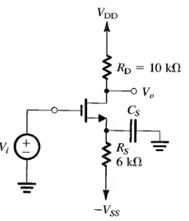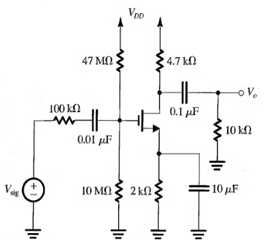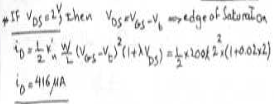# Test: Biasing In MOS Amplifier Circuit

## 10 Questions MCQ Test Electronic Devices | Test: Biasing In MOS Amplifier Circuit

Description
This mock test of Test: Biasing In MOS Amplifier Circuit for Electrical Engineering (EE) helps you for every Electrical Engineering (EE) entrance exam. This contains 10 Multiple Choice Questions for Electrical Engineering (EE) Test: Biasing In MOS Amplifier Circuit (mcq) to study with solutions a complete question bank. The solved questions answers in this Test: Biasing In MOS Amplifier Circuit quiz give you a good mix of easy questions and tough questions. Electrical Engineering (EE) students definitely take this Test: Biasing In MOS Amplifier Circuit exercise for a better result in the exam. You can find other Test: Biasing In MOS Amplifier Circuit extra questions, long questions & short questions for Electrical Engineering (EE) on EduRev as well by searching above.
QUESTION: 1

Solution:
QUESTION: 2

Solution:
QUESTION: 3

### (Q.3-Q.4) The amplifier in the figure shown below is biased to operate at ID = 1mA and gm = 1mA/V.Q. Find the midband gain.

Solution:
QUESTION: 4

Find the value of CS that places FL at 10Hz

Solution:
QUESTION: 5

(Q.5-Q.6) )In the NMOS transistor of the circuit shown below is biased to have gm = 1mA/V and r0 = 100 kΩ.Q. Find AM

Solution:
QUESTION: 6

Find fH if Cgs = 1 pF and Cgd = 0.2 pF.

Solution:
QUESTION: 7

(Q.7-Q.8) For a particular depletion mode NMOS device, Vt = -2V, kn W/L = 200 µA/V2 and λ = 0.02V-1. For VDS = 2V

Q. What is the drain current that flows

Solution:QUESTION: 8

What is the value of the drain current if both L and W are doubled?

Solution:
QUESTION: 9

(Q.9-Q.10) A depletion type N channel MOSFEt with knW/L = 2 mA/V2 and Vt = 3V has its source and gate grounded. For Vd = 0.1V and neglecting channel length modulating effect

Q. Find drain current.

Solution:
QUESTION: 10

In which region is the triode operating?

Solution: